# Texas Go Math Grade 7 Lesson 7.1 Answer Key Linear Relationships in the Form y = mx + b

Refer to our Texas Go Math Grade 7 Answer Key Pdf to score good marks in the exams. Test yourself by practicing the problems from Texas Go Math Grade 7 Lesson 7.1 Answer Key Linear Relationships in the Form y = mx + b.

## Texas Go Math Grade 7 Lesson 7.1 Answer Key Linear Relationships in the Form y = mx + b

Discovering Linear Relationships

Many real-world situations can be described by linear relationships.

Jodie pays $5 per ticket for a play and a one-time$2 convenience fee. The table shows the total cost for different numbers of tickets.A. Describe a pattern for the row showing the number of tickets bought.
B. Describe the pattern for the row showing total cost.
C. Out of the total cost paid, how much does the actual ticket account for?

Reflect

Question 1.
How much more than $5 does Jodie pay for one ticket? What if she buys 5 tickets? Explain. Answer: Jodie pays$2 more for one ticket. If she buys 5 tickets she will pay also $2 more because only at the first payment is charged$ 2.

Lesson 7.1 Modeling Linear Relationships Answer Key Question 2.
Analyze Relationships Describe the total amount paid in dollars based on the number of tickets.
One ticket: 2 + 5= 7
Two tickets: 2 – 5 (2) = 12
Three tickets: 2 + 5 (3) = 17
Four tickets: 2 + 5 (4) = 22
Five tickets: 2 + 5 (5) = 27

One ticket cost $7. Two tickets cost$12.
Three tickets cost $17. Four tickets cost$ 22.
Five tickets $27. Example 1 A man’s shoe size is approximately 3 times his foot length in inches minus 22. Use a table to represent the relationship between foot length and shoe size. STEP 1: Make a table. Label the top row Foot length (in.) and the bottom row Shoe size. STEP 2: Enter some foot lengths in inches. Since ¡t is impossible to have a negative shoe size, pick a foot length that when multiplied by 3 will be greater than 22.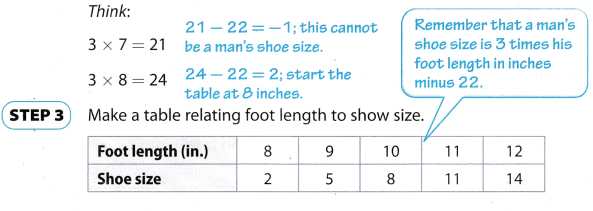Reflect Question 3. Analyze Relationships If someone had a foot length of 13 inches, how can you use the table to determine his shoe size? Answer: Multiply 13 by 3 and subtract 22 from that product 13 ∙ 3 = 39 39 – 22 = 17 Question 4. Critical Thinking Foot lengths do not have to be whole numbers. Give an example of a non-whole number foot length you could have chosen when filling in the table and find the approximate shoe size. What should a person do if their foot length does not correspond to a whole or half shoe size? Explain. Answer: For example: Foot Length of 12A in. 12.4 ∙ 3 – 22 = 37.2 – 22 = 15.2 therefore, it will be chosen shoe with a size of 15.5. Conclusion: If a number whose decimal is between 0 and 5 is obtained, then foot length corresponds to half shoe size, if a number whose decimal is between 5 and 9 is obtained then foot length corresponds to whole shoe size. Your Turn Go Math 7th Grade Answer Key Pdf Lesson 7.1 Question 5. Lea’s house is 350 meters from her friend’s house. Lea walks to her friend’s house at a constant rate of 50 meters per minute. Use a table to represent the relationship between time and the distance Lea has left to walk to her friend’s house.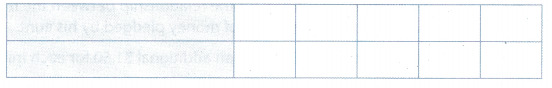Answer: At first divide 350 by 50.In this way, we are calculated how many minutes she has to walk to the friend’s house with a constant rate of 50 meters per minute. As we have five fields in the table for values of time and distance, divide 7 by 5.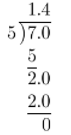The value of distance for each value of time in table we calculate as Distance = 50 ∙ timeShe has to walk 7 minutes. Example 2 Luis will participate in a walkathon for charity. He received a pledge from his aunt, and the table shows the relationshipbetween the miles walked by Luis and the amount his aunt pledged.Use the table to give a verbal description of the relationship between miles walked and amount pledged.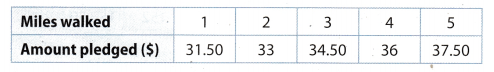STEP 1: Look for patterns in the different values for miles walked and amount pledged.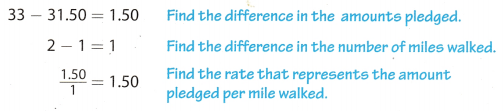In the table, each value for the number of miles walked is 1 greater than the previous one, and each amount pledged is$1.50 greater than the previous one.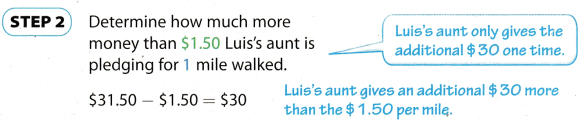STEP 3: Give a verbal description for the relationship between the miles walked by Luis and amount of money pledged by his aunt.
Luis’s aunt pledged $30 plus an additional$1.50 for each mile he walks.

Reflect

Question 6.
Make a Prediction How could you find the amount pledged by Luis’s aunt if Luis walks 7 miles? What is the amount pledged?
If Luis walks 7 miles the amount of pledged will be:
37.5 + 1.5 (2)
= $40.5 If Luis walks 7 miles the amount of pledged will be$ 40.5.

What If…? Luis’s mother decides to also pledge $15 plus an additional$3 per mile. If Luis wants to earn the same amount from his mother and his aunt, how far must he walk? What is the amount he will earn?
For aunt: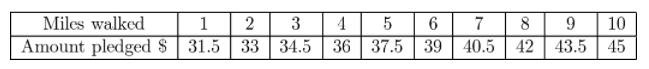For mother:He has to walk 10 miles to earn $45. Your Turn The relationship between the cost of an online advertisement for a movie and the number of times it is clicked on is shown in the table. Question 8. Use the table to give a verbal description of the relationship.Answer: To buy an online advertisement for a movie you will pay$150 plus an additional $0.5 for every tenth click. Question 9. What is the cost for the advertisement if it is clicked 1000 times? Answer: An online advertisement for a movie if it is clicked 1000 times will cost: 150 + 0.5 (1000) = 150 + 500 =$650
An online advertisement for a movie if it is clicked 1000 times will cost $650. Question 10. Is there a lower limit for the number of clicks? Is there an upper limit? Explain. Answer: For every ten clicks, we’re paying half a dollar, dividing that half-dollar difference from 10 to get a one-click cost. $$\frac{0.5}{10}$$ = 0.05 The upper limit does not exist because each click increases the price. Texas Go Math Grade 7 Lesson 7.1 Guided Practice Answer Key Question 1. The age of a cat 2 years or older can be approximately converted into human years by multiplying by 4 and adding 16. Use a table to represent the relationship between cat age and human years. (Example 1) Label the rows of the table. Choose numbers to represent the ages of the cat. Choose numbers that are 2 or greater, since the relationship described is only for cats 2 years or older. Complete the table by calculating the value for Human years based on the description.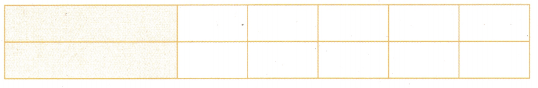Answer:Let X represent the age of a cat, and Y represent human years. To find human years we are solving the equation: X ∙ 4 + 16 = Y Go Math Grade 7 Lesson 7.1 Answer Key Question 2. The yearly cost of a community college based on the number of credits taken is shown in the table. Use the table to give a verbal description of the relationship between credits and cost. (Explore Activity and Example 2)STEP 1: Look for patterns in the different values for credits and cost. Each value for credits is _________ greater than the previous one, and each value for cost is _________ greater than the previous one. This means that 1 credit corresponds to _________ in cost. STEP 2: Determine how many more dollars than _________ that it costs to take 3 credits. It costs _________ – _________ = _________ more than _________ to take 3 credits. STEP 3: Give a verbal description for the relationship between credits and cost. The yearly cost of the community college is _________ plus _________ for each credit taken. Answer: Each value for credits is 3 greater than the previous one. Each value for cost is$75 greater than the previous one.
This mean one credit correspond to $25 in cost. Determine how many more dollars than$75 that it costs to take 3 credits.
It cost 175 – 75 = $100 more than$75 to take 3 credits.

The yearly cost of community college is $100 plus$25 for each credit taken.

Essential Question Check-In

Question 3.
When using tables and verbal descriptions to describe a linear relationship, why is it useful to convert from one to another?
Because in this way a connection is established and it is clearly explained how the connection has developed between them. It can be concluded quite a lot in case we know the value of one size and its relationship with another to accurately calculate the value of the other.

A teacher is making multiple copies of a 1 -page homework assignment. The time it takes her in seconds is 2 times the number of copies she makes plus 3.
Question 4.
What does the 3 represent in this scenario? What does the 2 represent?
This scenario we can represent with next equation:
y = 2 ∙ x + 3
Therefore when we look general form y = m ∙ x + b, in our equation m = 2 and b = 3.

What is the total number of seconds it takes for the teacher to make 1 copy? 2 copies? 3 copies? By how many seconds does the total time increase for each copy?
For one copy: x = 1
y = 2 (1) + 3
y = 5
For two copies: x = 2
y = 2 (2) + 3
y = 7
For three copies: x = 3
y = 2 (3) + 3
y = 9
For each subsequent copy, it takes two seconds more.

Question 6.
Represent Real-World Problems Represent the relationship between the number of copies made and time in seconds in the table below.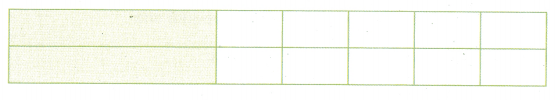The equation is: y = 2 ∙ x + 3, where y represent seconds and x represent number of copies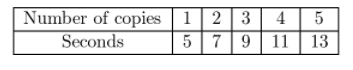Rosalee parks at a metered space that still has some time left. She adds some dimes to the meter. The table below represents the number of minutes left based on the number of dimes inserted into the meter.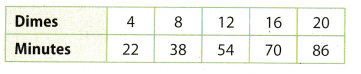Question 7.
How many minutes does 1 dime correspond to?
First find the difference between the adjacent values expressed in minutes
38 – 22 = 16
Divide the result by 4.
$$\frac{16}{4}$$ = 4
It takes 4 minutes for 1 dime.

Question 8.
Based on your answer to exercise 7, how many minutes should you receive for inserting 4 dimes?
You should receive 4 ∙ 4 = 16 minutes

Question 9.
Analyze Relationships Give a verbal description of the relationship between dimes and the number of minutes left on the meter.
Rosalee has 6 minutes left for parking plus an additional 4 minutes for each dime she adds.

Question 10.
Look at your answer for exercise 9. What does each of the numbers in the answer represent?
If we Look at the equation y = m ∙ x + b, for our example m = 16, b = 6, y represent how many minutes left for parking space and x represents how meny dimes are added.

The cost in dollars of a loaf of bread in a bakery is equal to 2 minus 0.25 times the number of days since it was baked.
Question 11.
What is different about this description compared to most of the other descriptions you have seen in this lesson?
The difference is that in our exercises it was mostly an increase for a certain value.
More precisely, we added a new value to the initial value, while in this case we deduct some value from the value.

Question 12.
Make a Conjecture Is there a point at which the linear relationship between days and dollars no longer makes sense?
Equation is: y = 2 – 0.25 ∙ x
8 days after being baked, the Linear relationship between days and dollars no longer makes sense, because:
y = 2 – 0.25 ∙ 8 = 2 – 2 = 0
y represent the cost in dollars of a loaf of bread and x represent a number of days since it was baked.

Represent Real-World Problems Represent the relationship between days and dollars in the table below.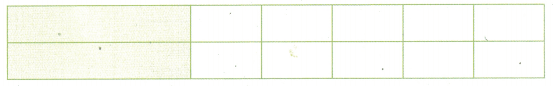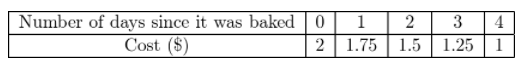y = 2 – 0.25 ∙ x

Question 14.
Find the number of days it will take the price to reach $0.25. Answer: In that case y = 0.25 Replace this in equation y = 2 – 0.25 ∙ x y = 2 – 0.25 ∙ x 0.25 = 2 – 0.25 ∙ x Subtract 2 from each side. 0.25 – 2 = 2 – 0.25 ∙ x – 2 – 1.75 = 0.25 ∙ x Divide both sides by -0.25 $$\frac{-1.75}{-0.25}$$ = x 7 = x It will take 7 days for a price to reach$ 0.25.

The relationship between the number of years since a tree was transplanted and its height in inches is shown in the table.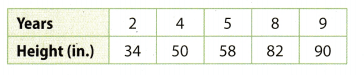Question 15.
What is different about this table compared to the other tables you have seen in this lesson?
In this table, there is no constant increase between the values for the variable x, while in each preceding case, we had the same increase for the value of the variable x. In this case, x represents the number of years.

Question 16.
Analyze Relationships Can you give a description of the relationship between the years since the tree was transplanted and its height in inches? If so, what is it?
The tree had a height of 18 in. before it was transplanted and plus an additional 8 in. for each year that follows.

H.O.T. Focus on Higher Order Thinking

Question 17.
Communicate Mathematical Ideas Suppose you are analyzing the relationship between time and distance given in a table, and there are 4 values for each quantity. You divide distance 2 minus distance 1 by time 2 minus time 1. You then divide distance 4 minus distance 3 by time 4 minus time-3 and get a different answer. What can you say about the relationship? Explain.
Persevere in Problem-Solving There is a linear relationship between a salesperson’s sales and her weekly income. If her sales are $200, her income is$500, and if her sales are $1,200, her income is$600. What is the relationship between sales and income?
y = $$\frac{1}{10}$$ ∙ x + 480
Critique Reasoning Molly orders necklace kits online. The cost of the necklace kits can be represented by a linear relationship. Molly’s order of 3 kits cost $12.50. Another order of 5 kits cost$17.50. Molly decides that the kits cost $5 each. Is she correct? Explain. Answer: Equation: y = $$\frac{5}{2}$$ ∙ x + 5 The procedure for obtaining this equation 5: First find the difference between the number of necklaces in these orders 5 – 3 = 2. Then find the difference between the prices 17.5 – 12.5 = 5 Divide this two values $$\frac{5}{2}$$ = 2.5 That much costs one necklace kits The kits cost$ 2.5 each. Molly’s answer is not correct.